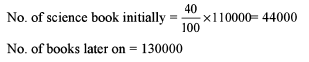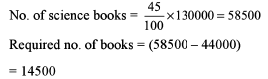Courses

# Test: Percentages- 4

## 15 Questions MCQ Test General Aptitude for GATE 2020 | Test: Percentages- 4

Description
This mock test of Test: Percentages- 4 for Quant helps you for every Quant entrance exam. This contains 15 Multiple Choice Questions for Quant Test: Percentages- 4 (mcq) to study with solutions a complete question bank. The solved questions answers in this Test: Percentages- 4 quiz give you a good mix of easy questions and tough questions. Quant students definitely take this Test: Percentages- 4 exercise for a better result in the exam. You can find other Test: Percentages- 4 extra questions, long questions & short questions for Quant on EduRev as well by searching above.
QUESTION: 1

### Because of the budget presented by Yashwant Sinha, the price of sugar increased by 40%. The Verma family reduced its consumption so that expenditure on sugar is up by 12%. If the total consumption of sugarbefore the rise in price was 50 kg. What is the present consumption of sugar (in kg)?

Solution:

Original price = Rs 100
Expenditure = Rs 50 x 100= Rs 5000 Expenditure after rise = Rs 5600 Then, 140 x x = 5600
X= 40

QUESTION: 2

### A’s income is reduced from Rs 75,000 to Rs 60,000, while B’s income for the same period is increased from Rs 60,000 to Rs 75,000. What percentage of decrease in A’s income is the increase in B ’s income?

Solution:

Decrease in A’s income = Rs 15000 Increase in B’s income = Rs 15000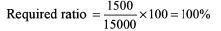QUESTION: 3

### Out of 51,600 candidates who appeared in an examination, 35% failed, while 15% passed with honours. What is the number of candidates who passed the examination, but failed to obtain honours, assuming that no student who has failed can obtain honours?

Solution: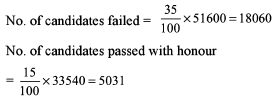Note: 15% of the remaining No. of students failed to obtain honour but got passed = 28509

QUESTION: 4

A locomotive engine runs at a speed of 50 km/h when no compartment is attached to it. For every new compartment that is attached to it, the speed of the train is reduced by 10% of the earlier speed. At most, how many compartments can be attached so that the train can cover a distance of 180 km in a maximum of 8 hours?

Solution:

Let n = number of components attached, Vn = speed of train when ‘n ’ compartm ents are attached

V0 = 50
V1 = 45

V2 = 40.5 and so on

At n = 7, we get the answer.

QUESTION: 5

If the price of an article rose by 25% every odd year and fell by 20% every even year, what would be the percentage change after 180 years?

Solution:

Let the original price = Rs 100

Price after 1st year = Rs 125

Price after 2nd year = Rs 100

Hence, after 180 year, there will be no change in the price

QUESTION: 6

In an examination, 80% students passed in philosophy and 70% students passed in maths. At the same time 15% failed in both the subjects. If390 students passed in both the subjects, how many students appeared in the examination?

Solution:

15% failed in both the subjects ⇒ 85% passed in at least one of the subjects.

So, percentage of students passing both the subjects = 80 + 70 - 85 = 65%
So, 65% = 390 ⇒ 100% = 600

QUESTION: 7

In the recent census report of Patna, it was found that 40% of total male adult population and 55% of total female adult population is married. What percentage of total adult population of Patna is married? (Polygamy is not in practice in Patna.)

Solution:

Let adult males = x and adult females = y then 0.55y = 0.4x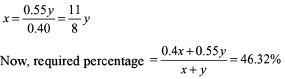QUESTION: 8

In an election, 10% of the voters on the voter’s list did not cast their votes and 60 voters cast their ballot papers blank. There were only two candidates. The winner was supported by 47% of all voters in the list and he got 308 votes more than his rival. The number of voters on the list were

Solution: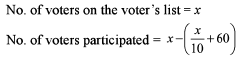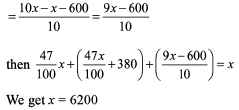QUESTION: 9

One-third of Mayank’s marks in history equals his marks in geography. If he obtained 180 marks in two subjects together, how much marks did he get in geography?

Solution:

x +y = 180           (1)
x - 3 y = 0            (2)
Solving the equations, we get x = 135, y = 45

QUESTION: 10

The entry fee in an exhibition was Rs 10. Later this was reduced by 25%, which increased the sale of tickets by 20%. Find the percentage increase in the number of visitors.

Solution:

10x 100=1000, 100 = no. of visitors Now, 7.5 x No. of visitors = 1200 No. of visitors =160

QUESTION: 11

An electrical contractor purchased a certain amount of wire, 10% of which was stolen. After using 85% of the remainder he had 47.25 m of wire left. How much wire did he purchase?

Solution: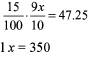QUESTION: 12

There were only two contestants A and B in the recent assembly election in Bihar at Raghopur constituency. B got 50% of the votes that A got. Had A got 200 votes less, there would have been a tie. What is the total number of votes polled?

Solution: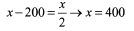A got 400 votes and B got 200 votes But is information 18 given regarding invalid votes.

QUESTION: 13

A is an alloy of tin and copper and B is an alloy of copper and zinc. A has 30% copper while B has 50% copper, x gm of A is mixed with 30 gins of B to form another alloy which has 45% copper. What is the value ofx?

Solution: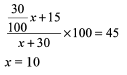QUESTION: 14

One litre of water is evaporated from 6 litres of solution containing 4% of sugar. The percentage of sugar in the remaining solution is

Solution: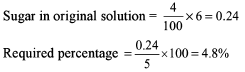QUESTION: 15

There are 1,10,000 books at Khuda Baksh Oriental Public Library, 40% of which are science books. It was decided to add 20,000 new books to the library. How many of the new books should be science booksin order to bring the percentage of science books in the library equal to 45%?

Solution: